## The Angular Momentum Eigenfunctions

The angular momentum eigenstates are eigenstates of two operators.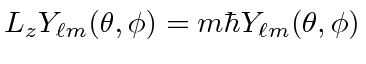All we know about the states are the two quantum numbersand. We have no additional knowledge about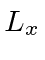andsince these operators don't commute with. The raising and lowering operators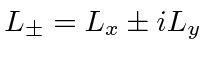raise or lower, leavingunchanged.The differential operators take some work to derive.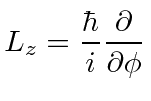Its easy to find functions that give the eigenvalue of.To find thedependence, we will use the fact that there are limits on. The state with maximummust give zero when raised.This gives us a differential equation for that state.The solution isCheck the solution.Its correct.

Here we should note that only the integer value ofwork for these solutions. If we were to use half-integers, the wave functions would not be single valued, for example atand. Even though the probability may be single valued, discontinuities in the amplitude would lead to infinities in the Schrödinger equation. We will find later that the half-integer angular momentum states are used for internal angular momentum (spin), for which noorcoordinates exist.

Therefore, the eigenstateis.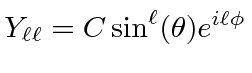We can compute the next state down by operating with.We can continue to lowerto get all of the eigenfunctions.

We call these eigenstates the Spherical Harmonics. The spherical harmonics are normalized.Since they are eigenfunctions of Hermitian operators, they are orthogonal.We will use the actual function in some problems.The spherical harmonics with negativecan be easily compute from those with positive.Any function ofandcan be expanded in the spherical harmonics.The spherical harmonics form a complete set.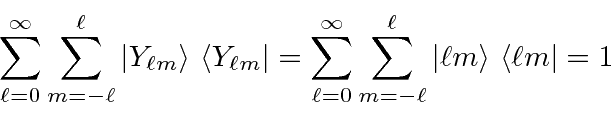When using bra-ket notation,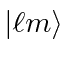is sufficient to identify the state.

The spherical harmonics are related to the Legendre polynomials which are functions of.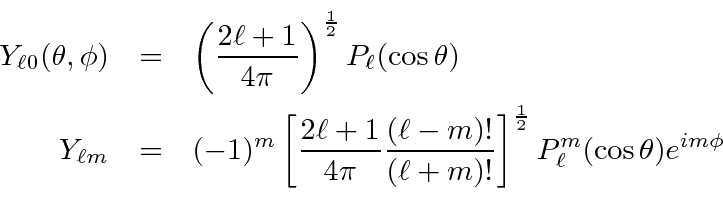Subsections
Jim Branson 2013-04-22